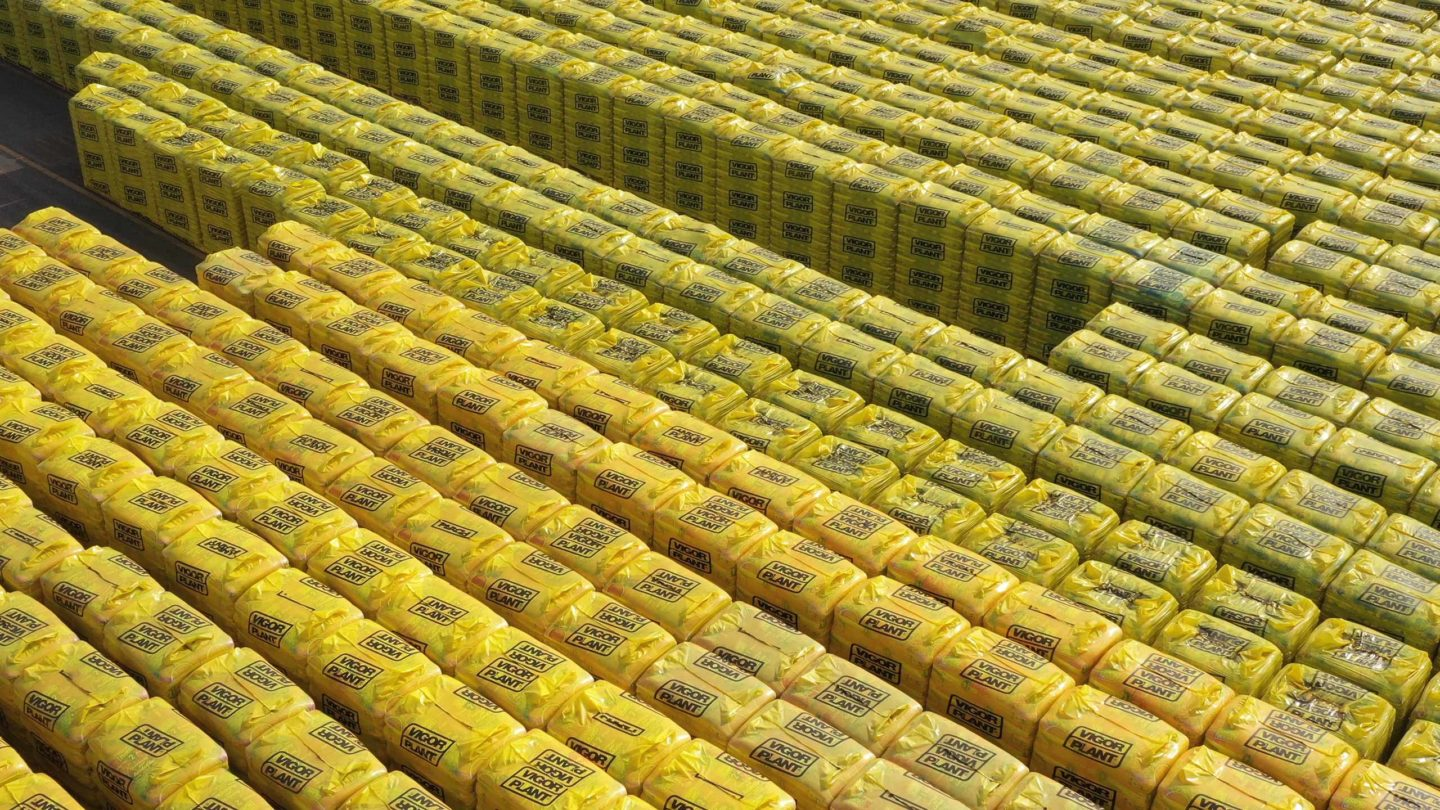# Volumetric control

Cultivation substrates are sold on a volumetric basis, but due to their nature as composite materials, i.e. consisting of a solid, a liquid and a gas phase, they are subject to volume changes resulting from the processes that take place between production and consumption, essentially, in the storage and transportation phases.

For this reason, some methods have been developed to allow the repeatable evaluation of the apparent volume of the material.

In Vigorplant, we use the method introduced by the European Union, EN 12580, and also incorporated in 2002 by the UNI Italian National Unification Body. We can therefore determine the quantity (volume) of substrate that is sold both in bulk and in packages.

Materials are weighed in a container of known volume, the apparent volume mass is then determined, and the volume is finally calculated from these same values.

#### Volumetric control

Here is how we calculate the volume, also known as the apparent density of the material.###### Disintegration grill

A meshed sieve of 20, 40 or 60 mm, depending on the material, is placed at no more than 50 mm above the collar.

###### Collar

It is rigid, of the same diameter as the measuring cylinder and with a height of 75±2 mm.

###### Measuring cylinder

A rigid container, with a volume of 20±0.4 l, with a height/diameter ratio between 0.9:1 and 1:1. The volume of the cylinder, V1, must be known with an accuracy of 10 ml at 20°C. (Please note that the specific gravity of the water at 20°C is 997.15g). It is therefore not necessary to make any pressure-related correction.

##### DA = ( M4 - M3 ) V1

M3 is the weight (mass) in grams of the measuring cylinder;
M4 is the weight (mass) in grams of the measuring cylinder and its content;
V1 is the volume in litres of the measuring cylinder.

FOR THE EVALUATION OF CUBE METERS, THE FOLLOWING PROCEDURE WILL BE USED: a 20-litre box of the product being sold is filled, making it pass through a screen with a 20, 40 or 60-mm mesh, depending on the type of product, without putting any mechanical pressure.

The net weight of the product contained in the 20-litre case, multiplied by 50 times, is equal to the weight of 1 m3.